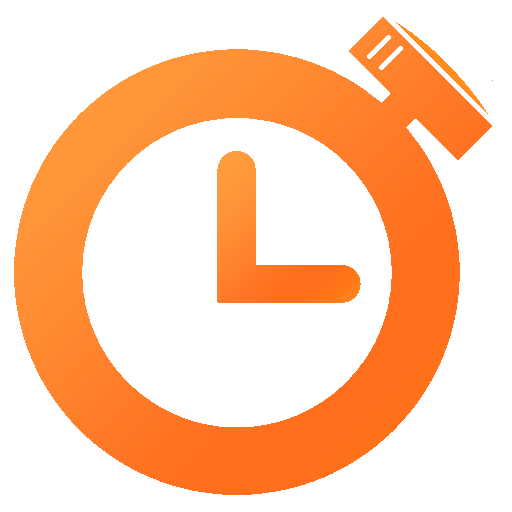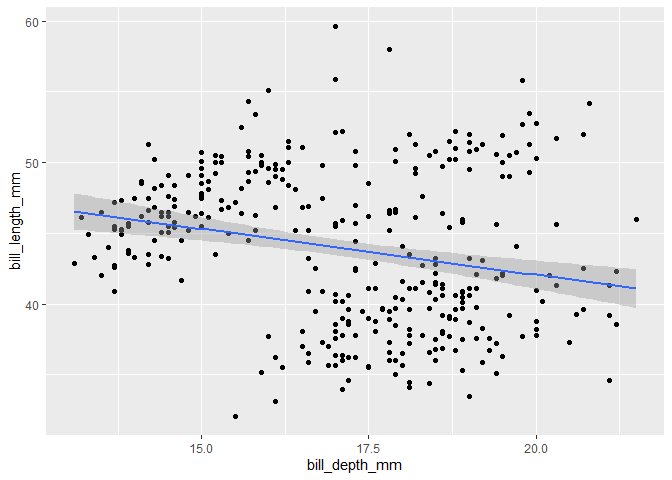# Linear Regression

In this module, you’ll learn to compute linear regression models in R. Feel free to skip review sections if you are confident in your knowledge.Time Estimates:Videos: 20 minReadings: 0 minActivities: 30 minCheck-ins: 3

## Review of Linear RegressionRequired Video: Review of Linear Regression IRecommended Video: Review of Linear Regression II

## Linear Regression in R

library(palmerpenguins)

penguins %>%
ggplot(aes(x = bill_depth_mm, y = bill_length_mm)) +
geom_point() +
stat_smooth(method = "lm")
## geom_smooth() using formula 'y ~ x'
## Warning: Removed 2 rows containing non-finite values (stat_smooth).
## Warning: Removed 2 rows containing missing values (geom_point).my_model <- penguins %>%
lm(bill_length_mm ~ bill_depth_mm, data = .)

my_model
##
## Call:
## lm(formula = bill_length_mm ~ bill_depth_mm, data = .)
##
## Coefficients:
##   (Intercept)  bill_depth_mm
##       55.0674        -0.6498
summary(my_model)
##
## Call:
## lm(formula = bill_length_mm ~ bill_depth_mm, data = .)
##
## Residuals:
##      Min       1Q   Median       3Q      Max
## -12.8949  -3.9042  -0.3772   3.6800  15.5798
##
## Coefficients:
##               Estimate Std. Error t value Pr(>|t|)
## (Intercept)    55.0674     2.5160  21.887  < 2e-16 ***
## bill_depth_mm  -0.6498     0.1457  -4.459 1.12e-05 ***
## ---
## Signif. codes:  0 '***' 0.001 '**' 0.01 '*' 0.05 '.' 0.1 ' ' 1
##
## Residual standard error: 5.314 on 340 degrees of freedom
##   (2 observations deleted due to missingness)
## Multiple R-squared:  0.05525,    Adjusted R-squared:  0.05247
## F-statistic: 19.88 on 1 and 340 DF,  p-value: 1.12e-05
broom::tidy(my_model)
## # A tibble: 2 x 5
##   term          estimate std.error statistic  p.value
##   <chr>            <dbl>     <dbl>     <dbl>    <dbl>
## 1 (Intercept)     55.1       2.52      21.9  6.91e-67
## 2 bill_depth_mm   -0.650     0.146     -4.46 1.12e- 5Check-In 1: Linear Regression

Question 1: Code

1. What is the data = . argument in the lm() function?

2. What happens if you switch the order of bill_length_mm and bill_depth_mm in the lm() formula?

3. What object type was returned by summary()? What about by tidy()?

Question 2: Interpreation

1. What is the equation for the regression line?

2. Penguin Bob has a bill that is 5mm deeper than Penguin Judy. How much longer do you expect Penguin Bob’s bill to be?

3. Is the relationship between bill length and bill depth statistically significant?

Question 3: A more complex model

Run the following code, and explore the results:

my_model_2 <- penguins %>%
lm(bill_length_mm ~ bill_depth_mm:species, data = .)

my_model_3 <- penguins %>%
lm(bill_length_mm ~ bill_depth_mm*species, data = .)
1. Make a plot illustrating my_model_2. (Hint: what needs to change in the aesthetic of the plot above?)

2. Which model of the three explains the most variance in the response variable?

3. Do the three species of penguin have the same average bill length? How do you know?

4. Do the three species of penguin have the same bill shape (i.e., the relationship between length and depth)? How do you know?